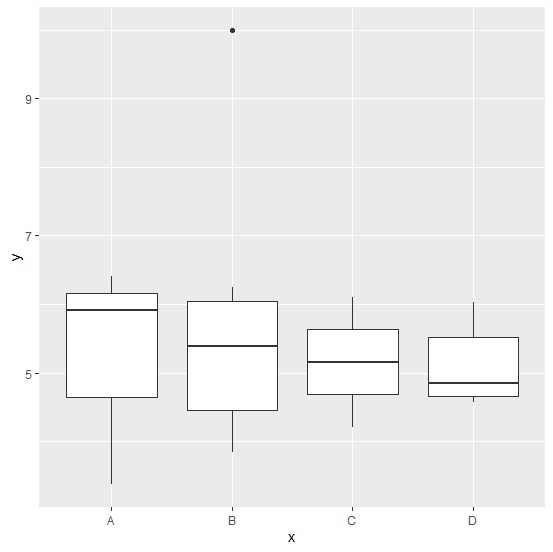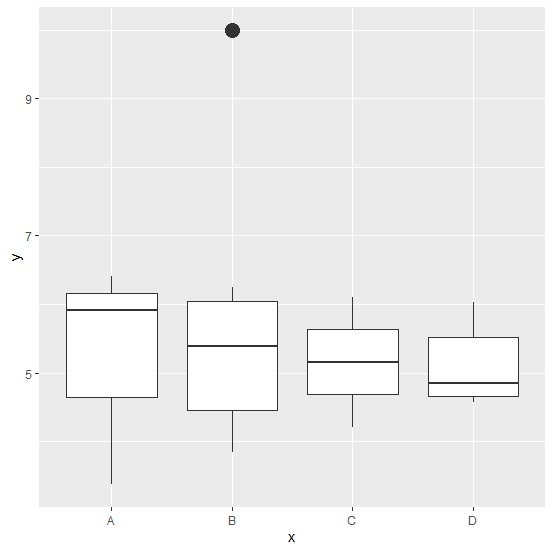# How to create a boxplot with outliers of larger size in R?

When we create a boxplot for a column of an R data frame that contains outlying values, the points for those values are smaller in size by default. If we want to increase the size for those outlying points then outlier.size argument can be used inside geom_boxplot function of ggplto2 package.

Consider the below data frame −

## Example

Live Demo

set.seed(1231)
x<-sample(LETTERS[1:4],20,replace=TRUE)
y<-c(rnorm(19,5,1),10)
df<-data.frame(x,y)
df

## output

x y
1 B 5.983562
2 A 4.571819
3 D 6.110019
4 A 4.074785
5 A 6.034136
6 B 5.351433
7 C 4.596340
8 C 3.375895
9 D 4.849060
10 A 4.723585
11 B 5.406556
12 A 6.254387
13 B 6.408786
14 C 5.386244
15 D 4.215608
16 A 4.576638
17 B 5.688985
18 B 3.839879
19 C 5.914120
20 B 10.000000

Loading ggplot2 package and creating boxplots for y values based on categories in x with default size of outliers −

## Example

library(ggplot2) ggplot(df,aes(x,y,col=x))+geom_boxplot()

## OutputCreating the boxplots with larger size of outlier −

## Example

ggplot(df,aes(x,y))+geom_boxplot(outlier.size=5)

## Output